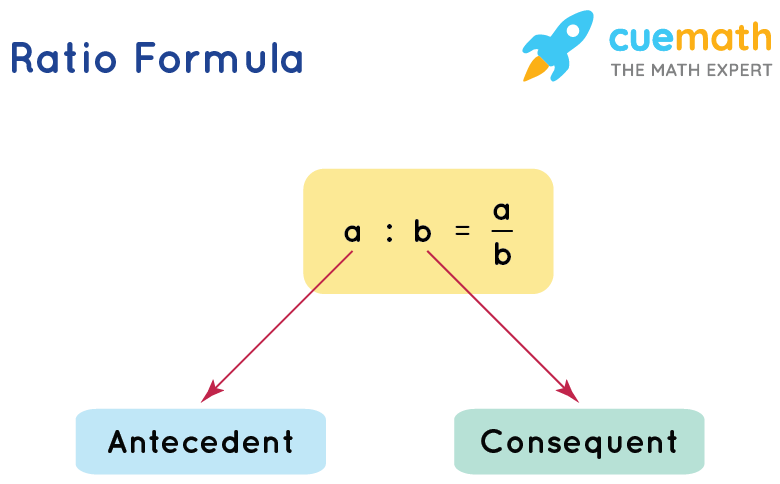# Ratio Formula

The ratio is defined as the relation between the quantities of two or more objects and it indicates the amount of one object contained in the other. We use the ratio formula while comparing the relationship between two numbers or quantities. The general form of representing a ratio of two quantities say a and b is

a:b

## What is the Ratio Formula?

The ratio formula for any two quantities say a and b is given as,

a:b = $$\dfrac{a}{b}$$Since a and b are individual amounts for two quantities, the total quantity combined is given as (a + b).

## Solved Examples Using Ratio Formula

### Example 1:

In a class of 70 students, 43 are girls and the remaining are boys.

Find the ratio of the total number of boys to the number of girls.

Solution:

To find: Ratio of the number of boys to the number of girls

Given:

Total number of students = 70

Number of girls = 43

Number of boys = Total number of students - Number of girls

= 27

Using Ratio Formula,

The ratio of number of boys to the number of girls = Number of boys: Number of girls = 27:43

### The ratio of x and y is 6:5. If x = 78, what is the value of y?

Solution:

To find: Value of x

Given:

Ratio of x to y = 6:5

Using Ratio Formula,

x:y = 6:5

\begin{align*} \dfrac{x}{y} &= \dfrac{6}{5} \\ \dfrac{78}{y} &= \dfrac{6}{5} \\ y &= \dfrac{5}{6} \times 78 \\ y &= 65 \end{align*}